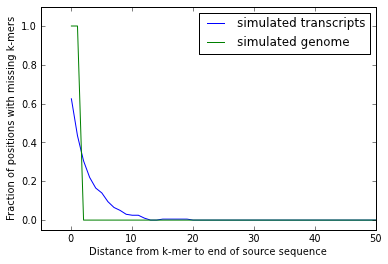Figure generation for

## "A Reference-Free Algorithm for Computational Normalization of Shotgun Sequencing Data"¶

In :
import numpy
num_points_to_plot = 50000  # affects size, rendering time for PDF plots.


# Figure 1¶

k-mer rank abundance plots

In :
rr1 = [ x.split() for x in open(datadir + 'read2.ranks') ]
rr1 = numpy.array([ (int(a), int(b)) for (a,b) in rr1 ])
rr1_x = [ a for (a, b) in rr1 ]
rr1_y = [ b for (a, b) in rr1 ]

rr2 = numpy.array([ (int(a), int(b)) for (a,b) in rr2 ])
rr2_x = [ a for (a, b) in rr2 ]
rr2_y = [ b for (a, b) in rr2 ]

rr3 = numpy.array([ (int(a), int(b)) for (a,b) in rr3 ])
rr3_x = [ a for (a, b) in rr3 ]
rr3_y = [ b for (a, b) in rr3 ]

In :
clf()
plot(rr1[:,0], rr1[:,1])
plot(rr2[:,0], rr2[:,1])
plot(rr3[:,0], rr3[:,1])
xlabel('k-mer rank')
ylabel('k-mer abundance')
legend(['no errors', 'single substitution error', 'multiple substitution errors'])
axis([0, 80, 0, 250])
savefig('diginorm-ranks.pdf')
show()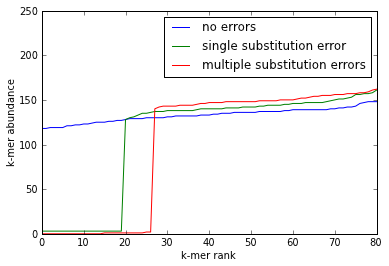# Figures 2 and 3¶

Correlation between median k-mer count and read coverage calculated from mapping, from both simulated data and genomic & transcriptomic data.

In :
import itertools, gzip
if filename.endswith('.gz'):
fp = gzip.open(filename)
else:
fp = open(filename)
if limit:
lines = [ line.split() for i, line in itertools.izip(range(limit), fp) ]
else:
lines = [ line.split() for line in fp ]
lines = [ (float(a), float(a)) for a in lines ]

print 'loaded %d lines' % len(lines)
return numpy.array(lines)

In :
genome_counts = load_cmp_file(datadir + 'genome-reads.fa.counts.cmp', limit=num_points_to_plot)

loaded 50000 lines

In :
clf()
axis([0, 300, 0, 300])
#axis(ymax=1000)

ylabel('read coverage by median k-mer count')
plot(genome_counts[:,0], genome_counts[:,1], 'b.', rasterized=True)

savefig('diginorm-sim-genome.pdf')
show()

c = numpy.corrcoef(genome_counts[:,0], genome_counts[:,1])[0,1]
print c**2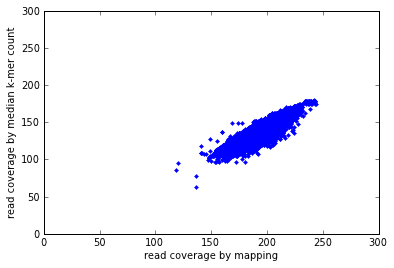0.786287931714

In :
ecoli_counts = load_cmp_file(datadir + 'ecoli_ref-5m.fastq.counts.cmp', limit=num_points_to_plot)

# remove points from Illumina crap; see text for details.
ecoli_counts = numpy.array([ (a,b) for (a,b) in ecoli_counts if b < 8000 ])

loaded 50000 lines

In :
clf()
#axis([0, 300, 0, 300])
#axis(xmax=200, ymax=200)
#axis(ymax=10500,ymin=10000)
ylabel('read coverage by median k-mer count')
plot(ecoli_counts[:,0], ecoli_counts[:,1], 'b.', rasterized=True)
savefig('diginorm-ecoli-genome.pdf')
show()

c = numpy.corrcoef(ecoli_counts[:,0], ecoli_counts[:,1])[0,1]
print c**2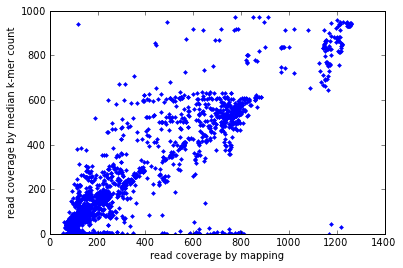0.798609411012

In :
transcript_counts = load_cmp_file(datadir + 'transcript-reads.fa.counts.cmp', limit=num_points_to_plot)

# remove points from Illumina crap; see text for details.
transcript_counts = numpy.array([ (a,b) for (a,b) in transcript_counts if b < 20000 ])

loaded 50000 lines

In :
clf()
#axis([0, 250, 0, 250])
ylabel('read coverage by median k-mer count')
loglog(transcript_counts[:,0], transcript_counts[:,1], 'b.', rasterized=True)

savefig('diginorm-sim-transcr.pdf')
show()

c = numpy.corrcoef(transcript_counts[:,0], transcript_counts[:,1])[0,1]
print 'linear r^2', c**2

c = numpy.corrcoef([ math.log(z) for z in transcript_counts[:,0] ], [ math.log(z) for z in transcript_counts[:,1] ])[0,1]
print 'log r^2', c**2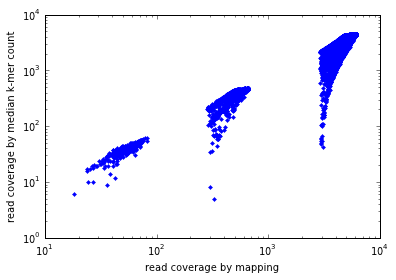linear r^2 0.931638777367
log r^2 0.956816045607

In :
mouse_counts = load_cmp_file(datadir + 'mouse-5m.fq.counts.cmp', limit=num_points_to_plot)

# remove points from Illumina crap; see text for details.
mouse_counts = numpy.array([ (a,b) for (a,b) in mouse_counts if b < 20000 ])

loaded 50000 lines

In :
clf()
#axis([0, 250, 0, 250])
ylabel('read coverage by median k-mer count')
loglog(mouse_counts[:,0], mouse_counts[:,1], 'b.', rasterized=True)

savefig('diginorm-mouse-transcr.pdf')
show()

c = numpy.corrcoef(mouse_counts[:,0], mouse_counts[:,1])[0,1]
print 'linear r^2', c**2

za = []
zb = []
for a, b in mouse_counts:
if a and b:
za.append(math.log(a))
zb.append(math.log(b))

c = numpy.corrcoef(za, zb)[0,1]
print 'log r^2', c**2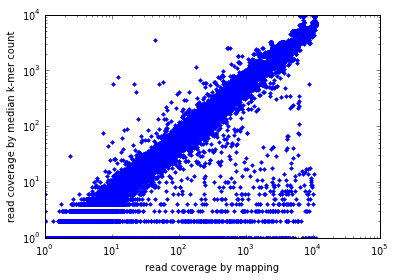linear r^2 0.904776248799
log r^2 0.872367665664


# Figure 4¶

In :
ecoli_cov = numpy.loadtxt(datadir + 'ecoli.rawreads.map.gz.cov')
ecoli_cov[:,1] /= sum(ecoli_cov[:,1])

staph_cov[:,1] /= sum(staph_cov[:,1])

sar_cov[:,1] /= sum(sar_cov[:,1])

In :
mapcov = numpy.loadtxt(datadir + 'genome-reads.fa.map.cov')


In :
mapcov[:,1] /= sum(mapcov[:,1])
keepcov[:,1] /= sum(keepcov[:,1])
ecolicov[:,1] /= sum(ecolicov[:,1])
ecoli20cov[:,1] /= sum(ecoli20cov[:,1])

transcript_cov[:,1] /= sum(transcript_cov[:,1])
mousecov[:,1] /= sum(mousecov[:,1])

transcript_keepcov[:,1] /= sum(transcript_keepcov[:,1])
mousekeepcov[:,1] /= sum(mousekeepcov[:,1])

In :
plot(ecoli_cov[:,0], ecoli_cov[:,1])
plot(staph_cov[:,0], staph_cov[:,1])
plot(sar_cov[:,0], sar_cov[:,1])
xlabel("Per-base coverage of reference genome")
ylabel("Fraction of total bases with that coverage")
legend(["E. coli", "S. aureus single cell MDA", "SAR324 single cell MDA"])
axis(xmax=2000)
savefig('/tmp/diginorm-coverage2-raw.pdf')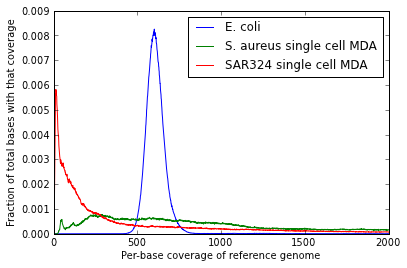In :
ecoli_kcov = numpy.loadtxt(datadir + 'ecoli.keep.rawreads.map.gz.cov')
ecoli_kcov[:,1] /= sum(ecoli_kcov[:,1])

staph_kcov[:,1] /= sum(staph_kcov[:,1])

sar_kcov[:,1] /= sum(sar_kcov[:,1])

In :
plot(ecoli_kcov[:,0], ecoli_kcov[:,1])
plot(staph_kcov[:,0], staph_kcov[:,1])
plot(sar_kcov[:,0], sar_kcov[:,1])
xlabel("Per-base coverage of reference genome")
ylabel("Fraction of total bases with that coverage")
legend(["E. coli", "S. aureus single cell MDA", "SAR324 single cell MDA"])
axis(xmax=100)
savefig('/tmp/diginorm-coverage2-dn.pdf')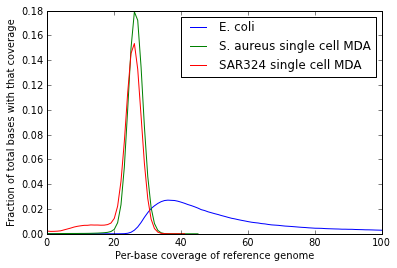# Figure 5¶

In :
fp = open(datadir + 'ecoli_ref.report')
report = [ x.split()[:2] for x in fp ]
report = [ (float(a) / 1e6, int(b)) for (a,b) in report[1:] ]
report = [ (a, b, float(b)/float(a)/1e6) for (a,b) in report if a ]
print report

(0.2, 199444, 0.99722)

In :
x = [ a for (a,b,c) in report ]
y = [ c for (a,b,c) in report ]

In :
clf()
axis([0, 30, 0, 1.0])
plot(x,y)
savefig('diginorm-accumulation.pdf')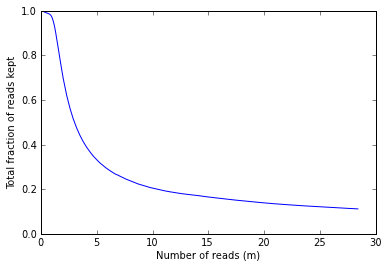# Figure 6 - end bias stuff¶

In :
def load_endbias(filename):
data = [ i.split() for i in open(filename) ]
x = [ int(a) for (a,_,b,_) in data ]
y = [ float(b) for (a,_,b,_) in data ]

return (x, y)


clf()